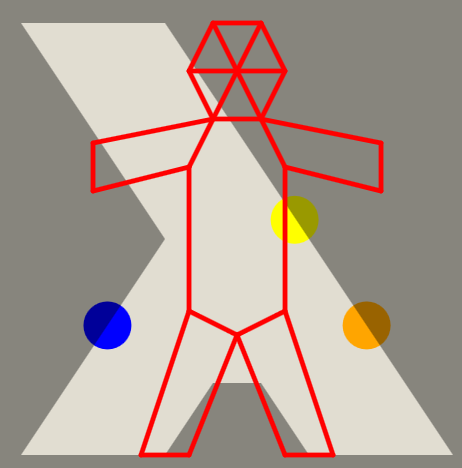# Problem 52308. ICFP2021 Hole-In-Wall: Calculate Score

The ICFP held its annual 3-day contest in July 2021 with Hole-In-Wall. Contest Specification.
The contest folds the figure in Red to fit within the hole shown in light greyThis Challenge is to evaluate the Score defined in the Specification when given the hole vertices in hxy and figure vertices in pxy. The hxy matrix is [N+1,2] where N is number of hole vertices. A repeat of the first vertex occurs for drawing the hole. The pxy matrix is [P,2] where P is the number of figure vertices. The pxy matrix will be valid and fit within the hole.
Score can be summarized as the sum of minimum square distances to the figure from each unique hole vertex. Score=calc_score(hxy,pxy)
These types of contests like to avoid non-integer calculations thus the distance squared calculation.
The ICFP 2021 Hole In Wall contest site has enabled a public user login to allow submissions. A login must be created to access all the problems and to submit solutions. Solutions are simple text files. Other challenges will show reading files, drawing figures, and producing submission files. To fully access the ICFP/Problems site use Register Team. Anyone can select Problems Page and then click problem numbers to see the puzzles and to download problem files.
The ICFP Contests page shows the annual contests back to 1998. The 2019 contest can be processed by Matlab. ICFP2019 Results Youtube gives a summary of this contest.

### Solution Stats

50.0% Correct | 50.0% Incorrect
Last Solution submitted on Nov 29, 2023

### Community Treasure Hunt

Find the treasures in MATLAB Central and discover how the community can help you!

Start Hunting!